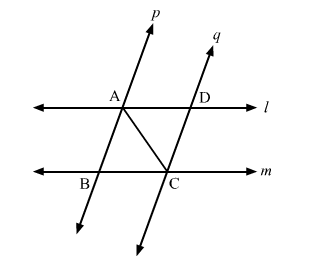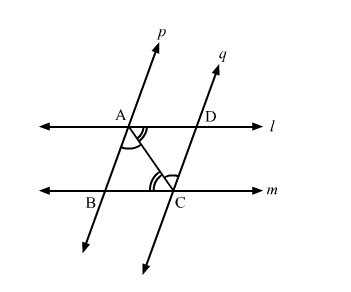# In the given figure, two parallel line l and m are intersected by two parallel lines p and q.

Question:

In the given figure, two parallel line l and m are intersected by two parallel lines p and q.
Show that ΔABC ≅ ΔCDA.Solution:Given: In the given figure, two parallel line l and m are intersected by two parallel lines p and q.

To prove: ΔABC ≅ ΔCDA

Proof:
In ΔABC and ΔCDA,

">BAC = ">DCA           (Alternate interior angles, p">q)
">BCA = ">DAC           (Alternate interior angles, l">m)
AC = CA                        (Common side)

"> By ASA congruence criteria,
ΔABC ≅ ΔCDA